## Randomize a list in Excel (Easy step-by-step tutorial)

Performing random sort is a quick and easy way to ensure that you end up with all cases turning up with equal probability. In this tutorial, we will talk about how to easily randomize, or shuffle a list in Excel in few steps.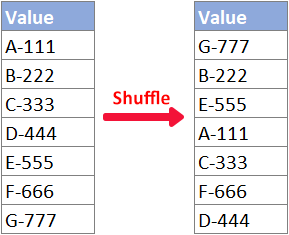#### Randomize a list with functions

In this section, I will teach you how to shuffle a list with built-in Excel functions.

##### Randomize a list with RAND function

Users of any Excel version can randomly sort their original dataset by using the RAND function with the help of Sort feature, please follow the steps below.

Step 1: Enter the RAND formula

Select the cell next to the top cell of the list you will randomize, input the below RAND formula, and press Enter.

``=RAND()``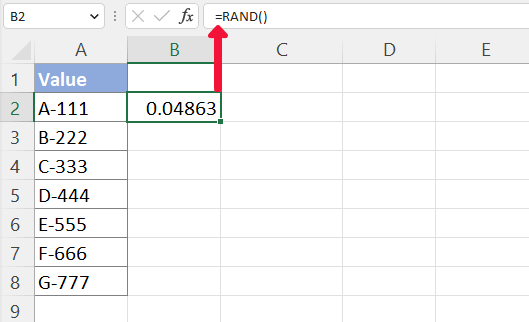Step 2: Fill the RAND formula to other cells

Double-click on the fill handle (the small green square in the lower-right corner) of the formula cell to apply the formula to below cells.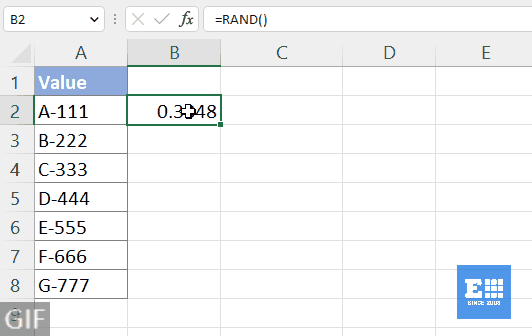Step 3: Sort the RAND results to shuffle the list

1. Select B2:B8, the list that contains the RAND formula.

2. Click on Sort & Filter > Sort Smallest to Largest in the Editing group on the Home tab.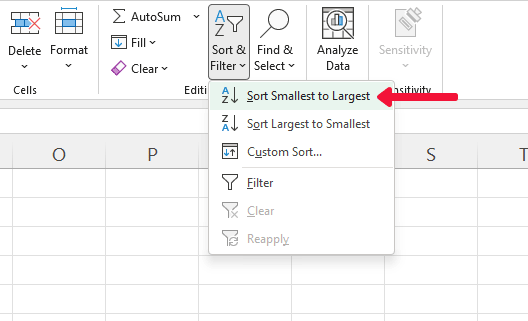3. In the pop-up Sort Warning dialog, select Expand the selection, and then click on Sort.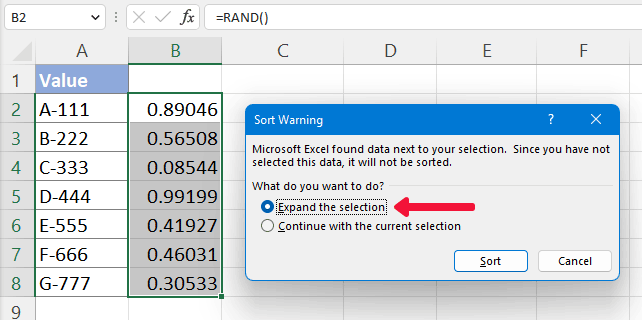Result

Now, you are all set! The list is randomized as shown below.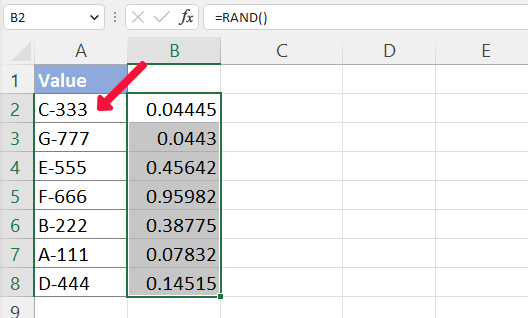Note: The Excel RAND function is volatile: It recalculates its result on every worksheet change. So, the numbers in column B changed instantly after sorting. If you want to shuffle the list again, repeat the above step 3. Otherwise, you could simply delete the column with the RAND function.
##### Randomize a list with RANDARRAY, SORTBY and ROWS functions (Excel 365/2021)

If you are users of newer Excel versions, for example, Excel for Microsoft 365 or Excel 2021, or Excel for the Web, the RANDARRAY, SORTBY and ROWS formula will help you quickly get a list shuffled at the position you specified.

Step 1: Enter the RANDARRAY, SORTBY and ROWS formula

Select a blank cell where you want to get the randomized result, type in the below formula (Note that you should change A2:A8 in below formula to the original list you want to randomize) and press Enter.

``=SORTBY(A2:A8,RANDARRAY(ROWS(A2:A8)))``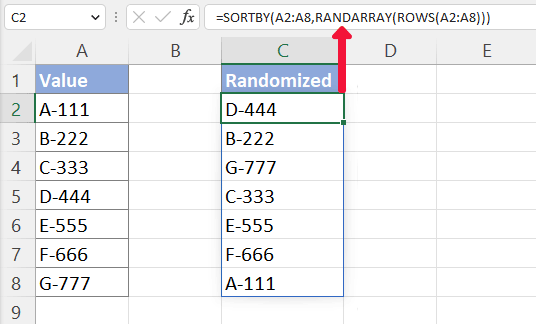Note:
• #SPILL errors are returned when the spill range (B2:B8 in this case) for the formula isn't blank.
• The formula is volatile: It recalculates its result on every worksheet change. If you want to shuffle the list again, press F9.
• The formula result (randomized list) is not editable. If you need to edit the randomized list, follow the next step.

(Optional) Step 2: Copy and paste the formula result to make it editable

If you only want to see a random result, you can skip this step. If you want to edit the randomly shuffled list, you should copy the formula result and paste values only:

1. Select the formula result and press Ctrl + C to copy it.

2. Right-click on a cell where you will paste the copied result. (You can also paste the value to its original location.)

3. Select the Paste Values Only option from right-clicking menu.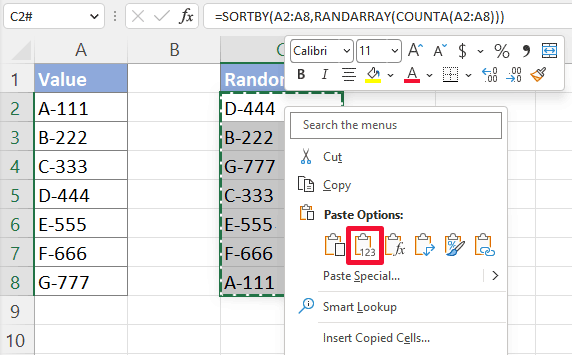Result

Now you will get a shuffled and editable list as shown below.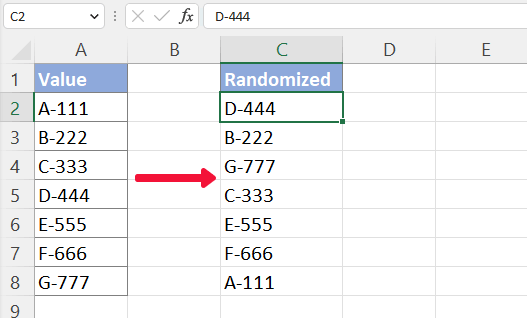#### Randomize a list in Excel with Kutools in 2 clicks

If you are tired of using formulas and intend to use the help of an add-in, Kutools for Excel’s Sort/Select Range Randomly feature can help you perform a random sort much easier with more options. Please so as follows:

First, select the list you will shuffle. And then select Kutools > Range > Sort/Select Range Randomly. In the pop-up Sort/Select Range Randomly dialog, select Entire rows, and then click on Ok.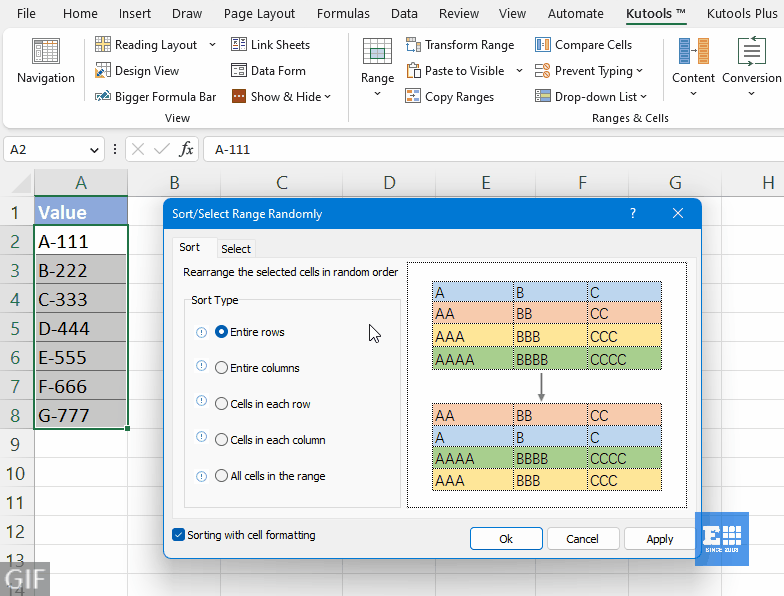Note:

#### Randomize a list with VBA

If you prefer to randomize a list with VBA method, please do as follows.

Note: You cannot undo after running a VBA macro. Therefore, make a copy of the list somewhere else in case you need the original data in the future.

Step 1: Select the list you will shuffle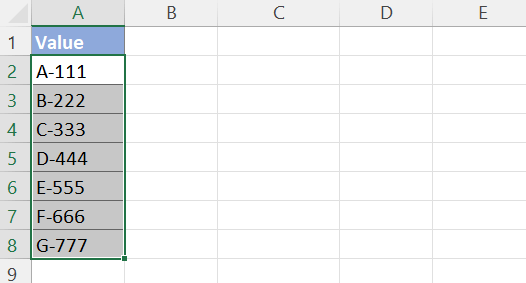Step 2: Copy VBA code to Module window

1. Press Ctrl + F11 to open the VBA editor, and then click Insert > Module to open a Module code window.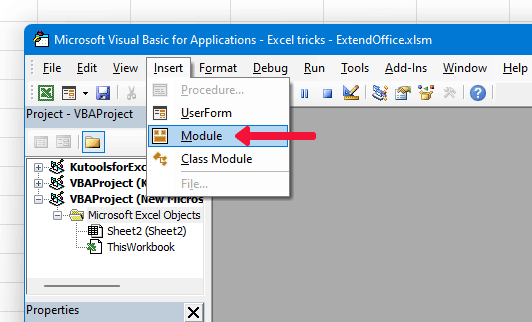2. Copy and paste the VBA code below to the opened Module window.

VBA code: Random sort a list

``````Sub RandomSort()
'Update by ExtendOffice
Dim xRg As Range
Dim xNum, xF, xI As Integer
Dim xWSh, xAWSh As Worksheet
On Error Resume Next
Application.ScreenUpdating = False
Set xAWSh = Application.ActiveSheet
Set xRg = ActiveWindow.RangeSelection
xNum = xRg.Count
For xF = xNum To 1 Step -1
xI = WorksheetFunction.RandBetween(1, xF)
xWSh.Range("A1").Value = xRg.Item(xI)
xRg.Item(xI) = xRg.Item(xF)
xRg.Item(xF) = xWSh.Range("A1")
Next
xWSh.Delete
Application.ScreenUpdating = True
End Sub``````

Step 3: Run the VBA code

In the code window, press F5 or click the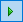button to run this code.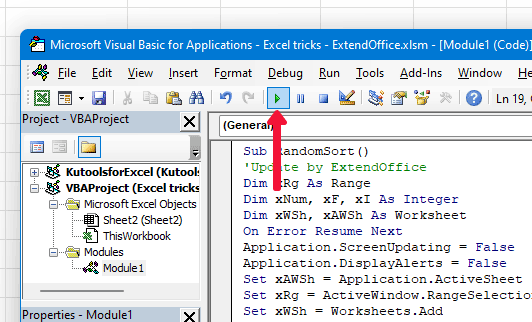Result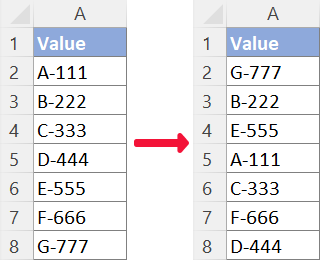Note: If you want to randomize the list again, repeat the above step 3.